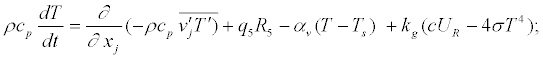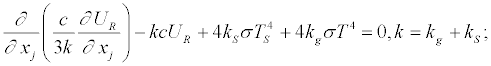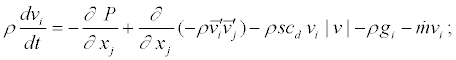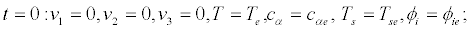Reach Us+44-7723-59-8358
All submissions of the EM system will be redirected to Online Manuscript Submission System. Authors are requested to submit articles directly to Online Manuscript Submission System of respective journal.

# Three Dimensional Mathematical Model of Crown Forest Fire Spread

Valeriy Perminov*

Tomsk Polytechnic University, Lenin Avenue, 30, Tomsk, 634050 Russia

Corresponding Author:
Valeriy Perminov
Tomsk Polytechnic University
Lenin Avenue, 30, Tomsk,634050 Russia
E-mail: [email protected]

Received date: 08/11/ 2015; Accepted date: 05/02/ 2016; Published date: 10/02/2016

Visit for more related articles at Research & Reviews: Journal of Ecology and Environmental Sciences

## Abstract

Mathematical model of forest fire was based on an analysis of known experimental data and using concept and methods from reactive media mechanics. In this paper the assignment and theoretical investigations of the problems of crown forest fire initiation in windy condition were carried out. The investigation takes in to account the mutual interaction of the forest fires and three dimensional atmosphere flows. The research is done by means of mathematical modeling of physical processes. It is based on numerical solution of Reynolds equations for chemical components and equations of energy conservation for gaseous and condensed phases. It is assumed that the forest during a forest fire can be modeled as a two-temperature multiphase non-deformable porous reactive medium. A discrete analog for the system of equations was obtained by means of the control volume method. The developed model of forest fire initiation and spreading would make it possible to obtain a detailed picture of the variation in the velocity, temperature and chemical species concentration fields with time. Mathematical model and the result of the calculation give an opportunity to evaluate critical conditions of the forest fire initiation and spread which allows applying the given model for of means for preventing fires.

#### Keywords

Mathematical model, Forest fire, Combustion.

#### Introduction

Analysis of the characteristics of forest fires reveals their dependence on the specific conditions under which the experiments were conducted, and their insufficient accuracy, which results from the fact that under actual conditions it is impossible to control the meteorological situation and the homogeneity of forest fuel layer. For this reason the physical modeling of forest fires must be combined with mathematical experiments using numerical methods and computers. The object of study in this case is not nature, but a mathematical model of a forest fire, which usually consists of a set of partial differential equations with appropriate initial and boundary conditions. Integral or functional equations can be used instead of differential. The deduction of a system of equations, and boundary and initial conditions, and the subsequent solution of the corresponding mathematical problem is usually called mathematical modeling of forest fires. Mathematical modeling possesses a number of advantages over physical experimentation, for example, ecological safety and greater economic efficiency. One of the objectives of these studies is the improvement of knowledge on the fundamental physical mechanisms that control forest fire initiation and spread. Considering that, natural investigations of these problems are merely impossible, methods of mathematical modeling are urgent. Mathematical model of forest fire was based on an analysis of known experimental data and using concept and methods from reactive media mechanics. Crown fires are initiated by convective and radiative heat transfer from surface fires. The first explanation of this process was given by Van Wagner . The theory proposed there depends on three simple crown properties: crown base height, bulk density and moisture content of forest fuel. Also crown fire initiation and hazard have been studied and modeled in detail [2-10]. The more complete mathematical model of forest fires is provided by Grishin et al. . Tomsk University [11-13]. In particular, this model which was obtained by Grishin  based on an analysis of known and original experimental data [11,14], and using concepts and methods from reactive media mechanics. The physical two-phase models used in [15,16] may be considered as a continuation and extension of the formulation proposed by Grishin [11-13]. However, the investigation of crown fires has been limited mainly to cases studied of forest fires initiation without take into account the mutual interaction of the forest fires and three dimensional atmosphere flows. This restriction has been removed in present setting of problem. Boundary layer of the atmosphere, forest canopy and the lower tier of the forest is considered as a single region.

#### Physical and Mathematical Formulation

The basic assumptions adopted during the deduction of equations, and boundary and initial conditions: 1) the forest represents a multi-phase, multistoried, spatially heterogeneous medium; 2) in the fire zone the forest is a porous-dispersed, two-temperature, single-velocity, reactive medium; 3) the forest canopy is supposed to be non - deformed medium (trunks, large branches, small twigs and needles), affects only the magnitude of the force of resistance in the equation of conservation of momentum in the gas phase, i.e., the medium is assumed to be quasi-solid (almost non-deformable during wind gusts); 4) let there be a so-called “ventilated” forest massif, in which the volume of fractions of condensed forest fuel phases, consisting of dry organic matter, water in liquid state, solid pyrolysis products, and ash, can be neglected compared to the volume fraction of gas phase (components of air and gaseous pyrolysis products); 5) the flow has a developed turbulent nature and molecular transfer is neglected; 6) gaseous phase density doesn’t depend on the pressure because of the low velocities of the flow in comparison with the velocity of the sound. Let the coordinate reference point x1, x2 , x3= 0 be situated at the centre of the surface forest fire source at the height of the roughness level, axis 0x1 directed parallel to the Earth’s surface to the right in the direction of the unperturbed wind speed, axis 0x2 directed perpendicular to 0x1 and axis 0x3 directed upward (Figure 1).

Using the results of [11-14] and known experimental data  we have the following sufficiently general equations, which define the state of the medium in the forest fire zone, written using tensor notation:(1)(2)(3)(4)(5)(6)(7)Reaction rates of these various contributions (pyrolysis, evaporation, combustion of coke and volatile combustible products of pyrolysis) are approximated by Arrhenius laws whose parameters (pre-exponential constant ki and activation energy Ei) are evaluated using data for mathematical models [11,13]. The system of equations (1)–(7) must be solved taking into account the initial and boundary conditions [11-14]:(8)(9)(10)(11)(12)(13)(14)

Here and aboveis the symbol of the total (substantial) derivative; αv is the coefficient of phase exchange; ρ - density of gas – dispersed phase, t is time; vi - the velocity components; T, TS, - temperatures of gas and solid phases,UR - density of radiation energy, k - coefficient of radiation attenuation, P - pressure; cp – constant pressure specific heat of the gas phase, cpi, ρi,φ i – specific heat, density and volume of fraction of condensed phase (1 – dry organic substance, 2 – moisture, 3 – condensed pyrolysis products, 4 – mineral part of forest fuel), Ri – the mass rates of chemical reactions, qi – thermal effects of chemical reactions; kg , kS - radiation absorption coefficients for gas and condensed phases; Te - the ambient temperature; cα - mass concentrations of α - component of gas - dispersed medium, index α=1,2,...,5, where 1 corresponds to the density of oxygen, 2 - to carbon monoxide CO, 3 - to carbon dioxide and inert components of air, 4 - to particles of black, 5 - to particles of smoke; R – universal gas constant; Mα , MC, and M molecular mass of α -components of the gas phase, carbon and air mixture; g is the gravity acceleration; Ve=Ve(x3) – logarithm profile of velocity on the inlet boundary; cd is an empirical coefficient of the resistance of the vegetation, s is the specific surface of the forest fuel in the given forest stratum, g – mass fraction of gas combustible products of pyrolysis, α4 and α5 – empirical constants. To define source terms which characterize inflow (outflow of mass) in a volume unit of the gas-dispersed phase were used the following formulae for the rate of formulation of the gas-dispersed mixturem, outflow of oxygen R51, changing carbon monoxide R52 , generation of black R54 and smoke particles R 5s .

Coefficients of multiphase (gas and solid phase) heat and mass exchange are definedHere α = Nuλ/dS – coefficient of heat exchange for sample of forest combustible material (for example needle), Nu – Nusselt number for cylinder, λ – coefficient of heat conductivity for pine needle; γ – parameter, which characterize relation between molecular masses of ambient and inflow gases . It is supposed that the optical properties of a medium are independent of radiation wavelength (the assumption that the medium is “grey”), and the so-called diffusion approximation for radiation flux density were used for a mathematical description of radiation transport during forest fires. The components of the tensor of turbulent stresses, as well as the turbulent fluxes of heat and mass are written in terms of the gradients of the average flow properties . It should be noted that this system of equations describes processes of transfer within the entire region of the forest massif, which includes the space between the underlying surface and the base of the forest canopy, the forest canopy and the space above it, while the appropriate components of the data base are used to calculate the specific properties of the various forest strata and the nearground layer of atmosphere. The thermodynamic, thermophysical and structural characteristics correspond to the forest fuels in the canopy of a different (for example pine forest [11-14]) type of forest.

#### Numerical Calculation and Results

The boundary-value problem (1)–(7) was solved numerically using control volume method and the SIMPLE like algorithm . The ignition source was turned off in computer program automatically when the fire began to propagate. Fields of temperature, velocity, component mass fractions, and volume fractions of phases were obtained for different instants of time. Figures 2a-g present the distribution for temperature of gas phase and velocities.

Figures 2 a-g present the distribution for temperature(a-t=7s, b-t=9s, c – t=14s, d- 16s, e – t=17s, f – t=21s, g – t=27 s.) and velocities. The isotherms are moved in the forest canopy and deformed by the action of wind. The results of calculation give an opportunity to evaluate critical condition of the forest fire spread, which allows applying the given model for preventing fires. It overestimates the crown forest fire spread that depends on crown properties: bulk density, moisture content of forest fuel and etc. The model proposed there gives a detailed picture of the change in the velocity, temperature and component concentration fields with time, and determine as well as the influence of different conditions on the crown forest fire spread.

#### Conclusion

The developed model of forest fire initiation and spreading would make it possible to obtain a detailed picture of the variation in the velocity, temperature and chemical species concentration fields with time. Mathematical model and the result of the calculation give an opportunity to evaluate critical conditions of the forest fire initiation and spread which allows applying the given model for of means for preventing fires.

#### References